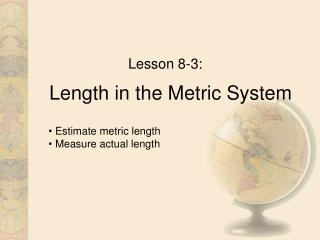DownloadDownload PresentationLength in the Metric System

# Length in the Metric System

Télécharger la présentation## Length in the Metric System

- - - - - - - - - - - - - - - - - - - - - - - - - - - E N D - - - - - - - - - - - - - - - - - - - - - - - - - - -
##### Presentation Transcript

1. Length in the Metric System Lesson 8-3: • Estimate metric length • Measure actual length

2. The Metric System • The metric system of measurement is a decimal system that uses prefixes to relate the sizes of units to standard units. • The standard unit of length is the meter. Metric Units of Length Unit ModelBenchmark 1 millimeter (mm) thickness of a dime 1mm ≈ 0.04 in 1 centimeter (cm) half the width of a penny 1cm ≈ 0.4 inch 1 meter (m) width of a doorway 1 m ≈ 1.1 yards 1 kilometer (km) six city blocks 1.6 km ≈ 1 mile

3. Length (distance) The standard unit is a meter. A meter is about the distance from the floor to the doorknob on a door. 1,000 meters make a kilometer. It is roughly 0.6 of a mile long. To run a kilometer, you would have to run around a track two and a half times. A centimeter is smaller than a meter. It takes 100 centimeters to make a meter. The thickness of a CD case is about 1 cm. A millimeter is very small. It takes 1,000 mm to make a meter. The thickness of a CD is about 1 mm.

4. Measuring Metric rulers show centimeters and millimeters. Line up the zero (or edge of the ruler if there is no zero) with the edge of what you’re measuring. The line is 5.3 cm long. You could also read it as 53 mm.

5. Practice TimeP 434 # 1-6